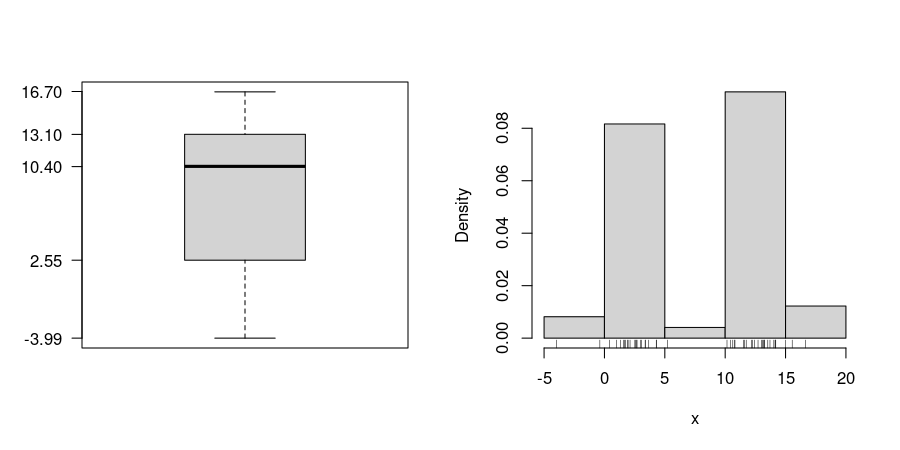## Exam 1

1. #### Question

For the 49 observations of the variable x in the data file boxhist.csv draw a histogram, a boxplot and a stripchart. Based on the graphics, answer the following questions or check the correct statements, respectively. (Comment: The tolerance for numeric answers is $±0.8$, the true/false statements are either about correct or clearly wrong.)
 The distribution is unimodal: true / false The distribution is: symmetric / right-skewed / left-skewed The boxplot shows outliers: true / false A quarter of the observations is smaller than: A quarter of the observations is greater than: Half of the observations are smaller than:

#### Solution1. False / True
2. True / False / False
3. False / True
4. 2.55
5. 13.1
6. 10.4